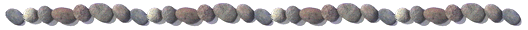Mathematica package BVPh 1.0

PublicationsInspirited by the general validity of the homotopy analysis method (HAM) in so many different fields and by the ability of “computing with functions instead of numbers” provided by computer algebra system such as Mathematica and Maple, a HAM-based Mathematica package BVPh (version 1.0) is developed for highly nonlinear boundary-value/eigenvalue equations.  The BVPh 1.0 is mainly valid for nonlinear ordinary differential equation with singularity, multiple solutions and/or multi-point boundary conditions in a finite or an infinite interval.  It is even valid for some nonlinear partial differential equations related to boundary-layer flows.  The aim is to develop a kind of analytic tool for as many nonlinear boundary-value problems (BVPs) as possible such that multiple solutions of some highly nonlinear BVPs can be conveniently found out, and that the infinite interval and singularity of governing equations and/or multi-point boundary conditions can be easily resolved.

Twelve examples for the use of the BVPh 1.0 are given in Part II of the book:

Homotopy Analysis Method in Nonlinear Differential Equations.  Higher Education Press & Springer, Beijing and Heidelberg, 2012.

As an open resource, the BVPh 1.0 can be free downloaded at the following hyperlinks.  The higher version of the BVPh will be issued in future.

Source Code:

Input Data Files of Examples:

Chapter 8:

Chapter 9:

Chapter 10:

Chapter 11:

Chapter 12: